Air Data Probes and Sensors for Small- and Mid-Sized Aircraft

Air Data Products

3D Displacement Sensors

Special Offers

Support

Measurement of Temperature on Aircraft

The following reference information was taken from: http://mtp.jpl.nasa.gov/notes/sat/sat.html

The relationship between static air temperature (SAT or Ts), and total air temperature (TAT or Tt), was given by:

(1)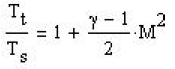where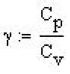is the ratio of the specific heats at constant pressure and volume, and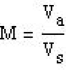is the Mach Number, which is the ratio of the aircraft's true air speed (TAS or va) , and the local velocity of sound vs .
Now the velocity of sound (to first order) depends only on the static air temperature (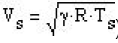), where all the quantities have been previously defined except the Gas Constant, R , which for dry air is 287.053 J kg-1 K -1 .

Measuring Temperature

We need to be clear about what is meant by the various temperatures:

• Static Air Temperature (SAT or Ts): This is the physical temperature of the air which the aircraft is flying through. It is also know as the outside air temperature (OAT); it is the temperature that we need to determine.
• Total Air Temperature (TAT or Tt): This is the temperature that would be measured by a probe if all of the kinetic energy of the air resulting from the aircraft's motion was absorbed. Because this is impossible, it can never be measured!
• Recovery Temperature (Tr): This is the temperature that the total air temperature is approximated by because of the incomplete recovery of the kinetic energy of the air by the temperature probe.
• Measured Temperature (Tm): As the name implies, this is the temperature that is actually measured by the aircraft's temperature probe. It differs from the recovery temperature, Tr, because of parasitic heating or cooling of the temperature sensor.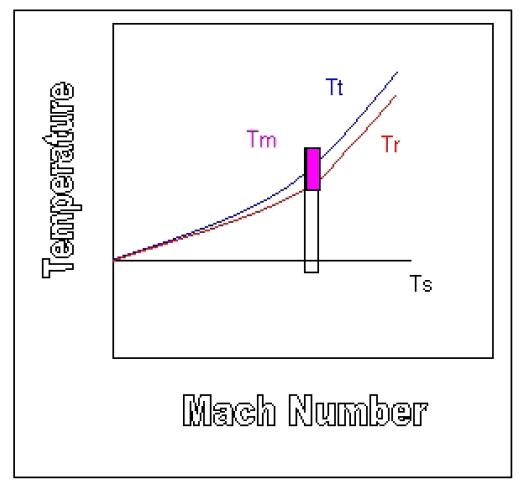The figure to the right shows the relationship between these temperatures. As might be expected, Tr is always less than Tt, and both these approach Ts as the Mach Number approaches zero. All of these temperatures can be be related to the flight speed, but Tm is more involved. As shown by the cyan and white vertical box, Tm may be higher or lower than Tt and Tr due to sensor design, location, and parasitics for any particular flight condition(that is, speed and attitude). In extreme case such as for vortex-producing probe designs or for severe weather conditions, Tm may actually be less than Ts. For a well-designed temperature probe, however, it is possible to achieve Tm > 0.995 Tt.

The parameter that relates the Tr to Tt and Ts is called the recovery factor ( r ), which is defined by:

(19)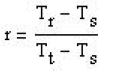Using this definition in Equation (1) we obtain:

(20)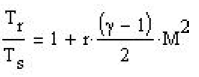For most total temperature sensors, the recovery factor varies with Mach Number. It is now more common to use the recovery correction defined by:

(21)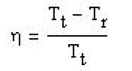Typically, the recovery correction varies with Mach Number below M = 1, but is constant for higher Mach Numbers. The recovery factor is related to the recovery correction by:

(22)Based on wind tunnel measurements for a specific total air temperature probe, a chart for the dependence of the recovery correction on Mach Number can be created. This in turn can be used in Equation (22) to obtain the recovery factor (r), which in turn, by assuming that Tm very closely equals Tr , allows Equation (20) to be solved for Ts. How well all this can be done depends on how well the total temperature probe is characterized for a number of other important effects:

Time Constant: How fast the probe responds depends on the heat capacity of the sensor parts

• Thermal Conduction: If the aircraft body is much warmer than the probe (for example, in the sunlight on the ground), heat may be transferred to the probe.
• Thermal Radiation: If the probe is very warm as it might be on the ground, it may radiate some of it's energy away, thus appearing cooler than it actually is.
• Airflow Direction: Although TAT probes are designed to be insensitive to the direction of the air stream entering the probe, there nevertheless remains some dependence, especially for large attitude excursions.
• Self-Heating: Some probes require a small constant current to flow through them in order to generate a voltage proportional to resistance (which depends on temperature). This current can increase the temperature of the sensing element.
• De-icing: Some probes have de-icing elements which when activated, can cause heat to flow toward the sensing element.

Generally the probe manufacturer will provide tables or equations which can be used in the Air Data Computer (ADC) to correct for these effects.

This document provides additional information on the subject of air temperature measurement on aircraft: http://www.grc.nasa.gov/WWW/BGH/stagtmp.html